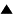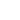# Homomorphism

The mathematical definition of group homomorphism is as follows. Let G1 and G2 be groups with the operations O1 and O2, respectively. A mapping M from G1 to G2 is a homomorphism if M(O1(x,y)) = O2(M(x),M(y)) for all x,y \in G1.

The difference from modeling is that in homomorphism the operations are defined on the pairs of elements from the group, while in modeling the operations are defined on pairs where the first element is a state of the world or the model, and the second -- an action, in the world or the model, respectively.

 HomeMetasystem Transition TheoryEpistemologyKnowledgeModel Up Prev.Next Down